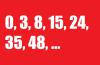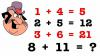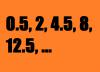BRAIN TEASERSBrain Teasers User Profile

# aysan saidie

rank
37
points
749
See full ranking list
short ranking list
 35 Mirjana Panković 992 36 Amir Shafa 900 37 aysan saidie 749 38 Sean Snow 630 39 Дејан Шкребић 610
 What number comes next? Look at the series (0, 3, 8, 15, 24, 35, 48, ?), determine the pattern, and find the value of the next number!Calculate 8+11 If 1+4=5, 2+5=12 and 3+6=21 then 8+11=?What is the next number in this series? Look at the series (0.5, 2, 4.5, 8, 12.5, ?), determine the pattern, and find the value of the next number!In 1921, Albert Einstein suggested the possibility of measuring the universe, which startled the audience, with his address Geometry and Expansion given at the Prussian Academy of Sciences in Berlin. Applying certain results of the relativity theory, he came to the conclusion that if the real velocities of the stars (as could be actually measured) were less than the calculated velocities, then it would prove that real gravitations' great distances were smaller than the gravitational distances demanded by the law of Newton. From such divergence, the finiteness of the universe could be proved indirectly, and it would even permit the estimation of its size.«